Computational & Technology Resources
an online resource for computational,
engineering & technology publications
Civil-Comp Proceedings
ISSN 1759-3433
CCP: 83
PROCEEDINGS OF THE EIGHTH INTERNATIONAL CONFERENCE ON COMPUTATIONAL STRUCTURES TECHNOLOGY
Edited by: B.H.V. Topping, G. Montero and R. Montenegro
Paper 215

J. Lógó, M. Ghaemi and A. Vásárhelyi

Department of Structural Mechanics, Budapest University of Technology and Economics, Hungary

Full Bibliographic Reference for this paper
, "Topology Optimization in the Case of Uncertain Loading Conditions", in B.H.V. Topping, G. Montero, R. Montenegro, (Editors), "Proceedings of the Eighth International Conference on Computational Structures Technology", Civil-Comp Press, Stirlingshire, UK, Paper 215, 2006. doi:10.4203/ccp.83.215
Keywords: topology optimization, stochastic programming, optimality criteria, compliance, optimal design, minimum volume design, probability.

Summary
In engineering practice uncertainties play a very important role but due to the complex nature of the stochastic calculation the designers usually apply deterministic data during the design process. The reason for the introduction of stochastic programming theory, more generally, probabilistic notation is to account in a more rational way for the fact that the precise strength of a structure is not known, among the constraints there are probabilistic inequalities and perhaps even more importantly, for the fact that the loadings applied to the structure are not known with any degree of precision. Loadings in particular are best described probabilistically. There is an extensive and expanding literature in this area. Even earlier significant publications were reported in this field. Vásárhelyi  created suitable numerical models for the stochastic analysis and design of panel structures. Marti  give fundamental results for either mathematical or mechanical aspects of stochastic optimization problems. Recently in the expanding field of optimal design the topology optimization is one of the mostly investigated fields of research. Generally, the papers mirror deterministic problems and only in recent publications have integrated the reliability analysis into a deterministic topology optimization problems by the introduction of the reliability constraint into the standard SIMP procedure.

The aim of this research is to introduce a new type of probability based topology design procedure. The standard deterministic mathematical programming problem determination is based on a minimum volume design procedure which is subjected to a compliance and side constraints. The loads and the supports are given in the usual way and the applied distribution functions and the mean values are given, too. Two problem groups are investigated. In first case the magnitudes of the concentrated forces (this is the loading) have uncertainties while in the second case the locations of the forces are described on probabilistic way. By the use of the Kataoka  model the numerical method is based on an optimality criteria procedure.

Introducing the deterministic problem an iterative technique (SIMP) and the connected numerical examples will be discussed briefly. The object of the design (ground structure) is a rectangular disk with given loading and support conditions. The material is linearly elastic. The design variables are the thickness of the finite elements. To obtain the correct optimal topology some filtering method (the ground elements are subdivided into further elements) has to be applied to avoid the so-called "checker-board pattern" . The optimization problem is to minimize the penalized weight of the structure which is subjected to a given compliance and side constraints as follows.

In case of loading uncertainties some parameters of the loading contain probabilistic quantities. They can be the magnitude, line of action or the point of application of the applied loads. From the mechanical point of view this information is a very different case but in numerical respect there is practically no difference between them. Due to these uncertainties the compliance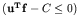condition has to be substituted by a probability value. If the joint normal distribution function, the mean values and the covariance matrix of the loads are given and denoted by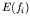,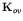, respectively, the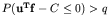condition can be substituted as follows :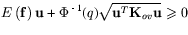(35)

In the proposed probabilistic topology optimization method: the minimum penalized weight design of the structure is subject to a compliance constraint which has uncertainties and side constraints. By the use of the above recommendation of Prekopa  the probabilistic expression (35) can be used as a constraint in the original problem. By the use of the first order optimality criteria a redesign formula of the stochastically constrained topology optimization problem can be derived.

References
1
A. Vásárhelyi "Limit Analysis and Optimal Plastic Design by Stochastic Programming with Uncertainties of Material Quality", Journal of Structural Mechanics, 15(2), 153-167, 1987.
2
K. Marti "Stochastic Optimization Methods", Springer-Verlag, Berlin-Heidelberg, 2005.
3
J. Lógó "New Type of Optimal Topologies by Iterative Method", Mechanics Based Design of Structures and Machines, 33(2), 149-172, 2005. doi:10.1081/SME-200067035
4